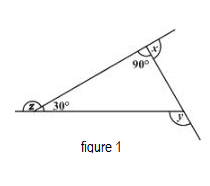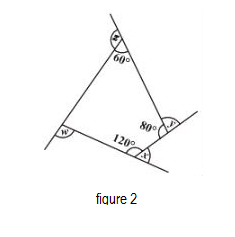QUESTION

# : Find the value of x + y + z in figure 1 and x + y + z + w in figure 2.Hint: Use the property sum of an exterior angle, and the corresponding interior angle of a polygon is $180{}^\circ$ . Also, use the property that the sum of all the interior angles of an n-sided polygon is $\left( n-2 \right)180{}^\circ$.Figure 1 is a Rights angled triangle. Now, using the property that sum of an exterior angle and the corresponding interior angle of a polygon is $180{}^\circ$ , we get
\begin{align} & z+30{}^\circ =180{}^\circ \\ & \Rightarrow z=150{}^\circ \\ \end{align}
\begin{align} & x+90{}^\circ =180{}^\circ \\ & \Rightarrow x=90{}^\circ \\ \end{align}
Now according to the exterior angle property of the triangle, the exterior angle of a triangle is the sum of the opposite interior angles.
$\therefore y=90{}^\circ +30{}^\circ =120{}^\circ$
Therefore, x + y + z = $360{}^\circ$ for figure 1.
Now let us move to figure 2.Figure 2 is a quadrilateral. Let the unknown interior angle of the quadrilateral be p.
Now, using the property that sum of an exterior angle and the corresponding interior angle of a polygon is $180{}^\circ$ , we get:
\begin{align} & z+60{}^\circ =180{}^\circ \\ & \Rightarrow z=120{}^\circ \\ \end{align}
\begin{align} & x+120{}^\circ =180{}^\circ \\ & \Rightarrow x=60{}^\circ \\ \end{align}
\begin{align} & y+80{}^\circ =180{}^\circ \\ & \Rightarrow y=100{}^\circ \\ \end{align}
We know that the sum of all the interior angles of a quadrilateral is equal to $360{}^\circ .$ Therefore, the unknown angle of the quadrilateral comes out to be:
\begin{align} & p+60{}^\circ +80{}^\circ +120{}^\circ =360{}^\circ \\ & \Rightarrow p=100{}^\circ \\ \end{align}
And using the property that sum of an exterior angle and the corresponding interior angle of a polygon is $180{}^\circ$ , we get
\begin{align} & p+w=180{}^\circ \\ & 100{}^\circ +w=180{}^\circ \\ & w=80{}^\circ \\ \end{align}
Therefore, we can conclude that x + y + z + w = $360{}^\circ$ in case of figure 2.

Note: The above question is based on the theorem that the sum of all the exterior angles of a polygon are $360{}^\circ$ . However, while solving such questions, it is a good practice to ensure that you are using all the data provided in the question.